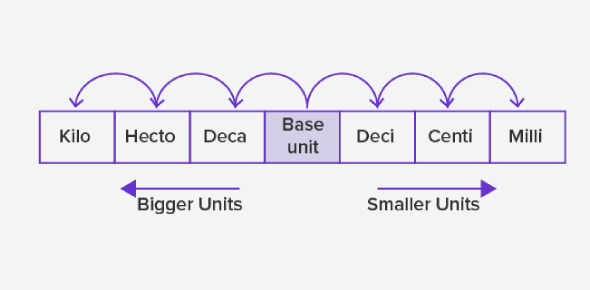Metric System Practice Quiz: Trivia

10 Questions | Total Attempts: 8222Settings.

• 1.
What is the base unit for measuring distance in the metric system?
• A.

Gram

• B.

Liter

• C.

Meter

• D.

Seconds

• 2.
What is the base unit for measuring mass in the metric system?
• A.

Kilogram

• B.

Liter

• C.

Meter

• D.

Seconds

• 3.
What is the base unit for measuring volume in the metric system?
• A.

Meter

• B.

Seconds

• C.

Liter

• D.

Gram

• 4.
What is the base unit for measuring time in the metric system?
• A.

Meter

• B.

Seconds

• C.

Liter

• D.

Gram

• 5.
Which scale is best for measuring the length of a ladybug?
• A.

Millimeters

• B.

Centimeters

• C.

Meters

• D.

Kilometers

• 6.
Which scale is best for measuring the length of a truck?
• A.

Millimeter

• B.

Centimeters

• C.

Meters

• D.

Kilometers

• 7.
How many grams in 4 kilograms?
• A.

4 grams

• B.

40 grams

• C.

400 grams

• D.

4000 grams

• 8.
How many kilograms in 8000 grams?
• A.

.0008 kilograms

• B.

8 kilograms

• C.

80 kilograms

• D.

800 kilograms

• 9.
Estimate the height of an average adult male in meters.
• A.

0.5 meters

• B.

1 meter

• C.

1.5 meters

• D.

2 meters

• E.

3 meters

• 10.
How many seconds in a day?
Related TopicsBack to top